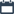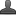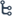## Tuesday, October 27, 2020

•October 27, 2020
••, ,
•No comments

## Important questions for 10th class Maths chapter-10 Mensuration,Mathematics questionnaire-x class

10.MENSURATION

Hi friends and my dear students! In this post, I have covered Important questions for 10th class maths chapter-5 Mensuration, Chapter wise previous year Questions. After Reading  Please do share it with your friends. Learn maths for All classes here

PRIORITY-I

2 MARK QUESTIONS:

1. The radius of conical tent is 7m and its height is 10m. Calculate the length of canvas used in the making the tent if width of canvas is 2m?

2. An oil drum is in the shape of a cylinder having the following dimensions. Diameter is 2m, and height is 7m. The painter charges Rs 3 per m2 to paint the drum. Find the total charges to be paid to the painter for 10 drums?

3. A sphere, a cylinder and a cone are of the same radius and same height. Find the ratio of their curved surface areas?

4. A company wanted to manufacture 1000 hemi spherical basins from a thin steel sheet. The radius of the hemi spherical basin is 21cm. Find the required area of steel sheet to manufacture the above hemi spherical basins?

5. A right circular cylinder has base radius 14cm, and height 21cm. find

i. area of base or area of each end

ii. Curved surface area

iii. Total surface area and

iv. Volume of the right circular cylinder?

6. Find the volume and surface area of a sphere of radius 2.1cm?

7. Find the volume and total surface area of a hemi sphere of radius 3.5cm?

8. Find the volume of right circular cone with radius 6cm and height 7cm?

9. The lateral surface area of a cylinder is equal to the curved surface area of a cone. If the radius be the same, find the ratio of the height of the cylinder and slant height of the cone?

10. A joker cap is in the form of right circular cone whose base radius is 7cm and height is 24cm. find the area of the sheet required to make 10 such caps?

11. A cylinder and a cone have bases of equal radii and are equal heights. Show that their volumes are in the ratio 3:1?

12. A heap of rice is in the form of a cone of diameter 12m, and height is 8m. Find its volume? How much canvas cloth is required to cover the heap?

13. Find the volume of the largest right circular cone that can be cut out of a cube whose edge is 7cm?

14. A metallic sphere of radius 4.2cm. is melted and recast into the shape of a cylinder of radius 6cm. find the height of the cylinder?

15. Metallic spheres of radius 6cm, 8 cm, and 10cm respectively are melted to form a single solid sphere. Find the radius of the resulting sphere?

4 MARKS QUESTIONS

1. A medicine capsule in the shape of a cylinder with two hemi spheres stuck to each of its ends. The length of the capsule is 14mm and the width is 5mm. find its surface area?

2. A toy is in the form of a cone mounted on a hemisphere the diameter of the base and the height of the cone are 6 cm and 4 cm respectively. Determine the surface area of the joy

3. Two cubes each of volume 64cm3 are joined end to end together. Find the surface area of the resulting cuboid?

4. A cylindrical container is filled with ice cream whose diameter is 12cm and height is 15cm the whole ice cream is distributed to 10 children in equal cones having hemispherical tops. If the height of the conical portion is twice the diameter of its base find the diameter of the ice cream cone

Also Check

## Important questions for 10th class maths chapter-5 Quadratic-equationsImportant questions for 10th class maths chapter-1 Real numbersImportant questions for 10th class maths chapter-4 LINEAR EQUATIONSImportant questions for 10th class maths chapter-3 polynomial5. A solid metallic sphere of diameter 28cm is melted and recast into a number of smaller cones each of diameter 4 2/3cm and height 3cm. find the number of cones so formed

6. A solid consisting of a right circular cone standing on a hemisphere is placed upright in a right circular cylinder full of water and touches the bottom find the volume of water left in the cylinder given that the radius of the cylinder is 3cm and its heights in 6cm. the radius of the hemisphere is 2cm and the height of the cone is 4cm

7. A solid toy is in the form of a right circular cylinder with hemi spherical shape at one end and a cone at the other end. Their common diameter is 4.2cm and the height

8. A women self help group (DWACRA) is supplied to a rectangular solid of wax with diameters 66cm,42cm, 21cm to prepare cylindrical candles each 4.2cm in diameter and 2.8cm of height. Find the number of candles?

8. A women self help group (DWACRA) is supplied to a rectangular solid of wax with diameters 66cm,42cm, 21cm to prepare cylindrical candles each 4.2cm in diameter and 2.8cm of height. Find the number of candles?

9. A hemispherical bowl of internal radius 15 cm. contains a liquid. The liquid is to be filled into cylindrical bottles of diameter 5 cm. and height 6 cm. How many bottles are necessary to empty the bowl?

10. A 20m deep well with diameter 7m. is dug and the earth from digging is evenly spread out to form a platform 22m. by 14m. Find the height of the platform.

11. An iron pillar consists of cylindrical portion of 2.8 m height and 20cm in diameter and a cone of 42cm height surmounting it. Find the we3ight of the pillar if 1 cm3 of iron weights 7.5g

PRIORITY II:

2 MARK QUESTIONS:

1. A cone of height 24cm and radius of base 6cm is made up of modeling clay. A child moulds it in the form of a sphere find the radius of the sphere.

2. The diameters of the internal and external surface of a hollow hemispherical shell are 6cm and 10cm respectively. It is melted and recast into a cylinder of diameter 14cm find the height of the cylinder.

3. The diameter of a metallic sphere is 6cm it is melted and drawn into a long wire having a circular cross section of diameter as 0.2cm find the length of the wire.

4. How many silver coins 1.75 cm in diameter and thickness 2mm need to be melted to form a cuboid of dimensions 5.5 cm x10cm x3.5cm?

5. The curved surface area of a cone is 4070cm2 and its diameter is 70cm what is its slant height?

6. A cubical box has each edge 10cm and another cuboid box has dimensions 12.5cm x 10cm x 8cm which box has grater surface area and how much?

7. A sphere is inscribed in a cylinder such that diameter of the sphere is equal to the height of the cylinder is the surface area of the sphere equal to the curved surface area of the cylinder? If yes explain how?

8. Express the volume of a sphere in terms of diameter‘d’?

9. In what ratio are the volumes of a cylinder a cone and a sphere if each has the same diameter and the same height?

10. By which multiplying the surface area of a sphere of radius ‘r’ the volume of sphere can be obtained?

11. Draw a solid shape combining more than two solid objects

12. If the dimensions of a cuboid are l, b and h respectively then express the length of the longest pole can be placed inside of it in terms of l, b and h?

13. Write the principle of T.S.A of cuboid and write nomenclature

14. If the ratio of volumes of two sphere is 64:27 find the ration of their radii

15. A cone is on a hemisphere whose combined diameter is 14cm if height of the cone is 7cm then find total surface area?

16. The volume and surface area of combined object is given as [1/3πr2h,2πr2] draw the suitable figure to it.

17. The radius of two cones are equal and the ratio of their slant heights are in the ration 3:2 then find the ratio of their curved surface area

18. If one liter of water to fill in a cube shape tin what are measurements requires of cube (in cms) explain

19. T.S.A of sphere is 4 πr2but T.S.A of hemisphere is 3 πr2 insted of 2 πr2 how do you justify write the LSA formula of cone and define the terms in it

20. The diameter of a metallic sphere is 6cm it is melted and drawn a wire having diameter of the cross section as 0.2 cm find the length of the wire.

21. A rectangular solid of wax with diameters 66cm, 42cm, 21cm is used to prepare 75 cylindrical candles having diameter 2.1cm height 2.8cm it is true? Support your answer?

22. A tap is hemisphere over a cone the common radius is 7cm height of the conical part is 24cm find the TSA and volume of the top.